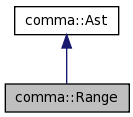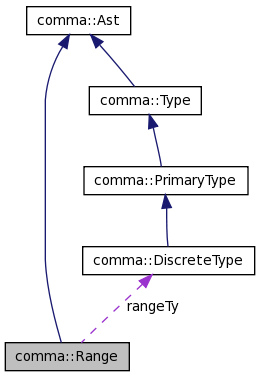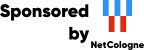## Welcome to the NetCologne GmbH open source mirroring service!

This machine mirrors various open-source projects. 20 Gbit/s uplink.

If there are any issues or you want another project mirrored, please contact mirror-service -=AT=- netcologne DOT de !

Comma: comma::Range Class Reference

# comma::Range Class Reference

The Range class represents a subset of values belonging to some scalar type. More...

`#include <Range.h>`

Inheritance diagram for comma::Range:[legend]
Collaboration diagram for comma::Range:[legend]

List of all members.

## Public Member Functions

Range (Expr *lower, Expr *upper, DiscreteType *type=0)
Location getLowerLocation () const
Returns the location of the lower bound of this range.
Location getUpperLocation () const
Returns the location of the upper bound of this range.
bool hasType () const
Returns true if this range has a type associated with it.
void setType (DiscreteType *type)
void setLowerBound (Expr *expr)
Resets the lower bound of this range.
void setUpperBound (Expr *expr)
Resets the upper bound of this range.
bool hasStaticLowerBound () const
Returns true if the lower bound is static.
bool hasStaticUpperBound () const
Returns true if the upper bound is static.
bool isStatic () const
const llvm::APInt & getStaticLowerBound () const
If this Range has a static lower bound, retrieve the value as an APInt.
const llvm::APInt & getStaticUpperBound () const
If this Range has a static upper bound, retrieve the value as an APInt.
bool isNull () const
bool contains (const llvm::APInt &value) const
uint64_t length () const

## Static Public Member Functions

static bool classof (const Range *node)
Support isa and dyn_cast.
static bool classof (const Ast *node)

DiscreteTypegetType ()
Returns the type of this range.
const DiscreteTypegetType () const
Returns the type of this range.
ExprgetLowerBound ()
Returns the expression defining the lower bound.
const ExprgetLowerBound () const
Returns the type of this range.
ExprgetUpperBound ()
Returns the expression defining the upper bound.
const ExprgetUpperBound () const
Returns the type of this range.

## Detailed Description

The Range class represents a subset of values belonging to some scalar type.

Definition at line 29 of file Range.h.

## Constructor & Destructor Documentation

 Range::Range ( Expr * lower, Expr * upper, DiscreteType * type = `0` )

Constructs a Range given expressions for the lower and upper bounds and an overall type for the range.

A range can be constructed without specifying a type. However, without a type this class cannot compute a uniform reprepresentation for any static bounds. More precisely, without a type, static bounds may have inconsistent bit widths. Therefore, it is advisable to resolve the type of the range before performing calculations using any static bound expressions.

hasType()
setType()

Definition at line 20 of file Range.cpp.

## Member Function Documentation

 static bool comma::Range::classof ( const Ast * node ) ` [inline, static]`

Definition at line 129 of file Range.h.

 static bool comma::Range::classof ( const Range * node ) ` [inline, static]`

Support isa and dyn_cast.

Reimplemented from comma::Ast.

Definition at line 128 of file Range.h.

 bool Range::contains ( const llvm::APInt & value ) const

Returns true if it is known that this range contains the given value.

This Range must be static for this function to return true.

Definition at line 110 of file Range.cpp.

 const Expr* comma::Range::getLowerBound ( ) const` [inline]`

Returns the type of this range.

Definition at line 72 of file Range.h.

 Expr* comma::Range::getLowerBound ( ) ` [inline]`

Returns the expression defining the lower bound.

Definition at line 71 of file Range.h.

 Location Range::getLowerLocation ( ) const

Returns the location of the lower bound of this range.

Definition at line 29 of file Range.cpp.

 const llvm::APInt& comma::Range::getStaticLowerBound ( ) const` [inline]`

If this Range has a static lower bound, retrieve the value as an APInt.

Definition at line 102 of file Range.h.

 const llvm::APInt& comma::Range::getStaticUpperBound ( ) const` [inline]`

If this Range has a static upper bound, retrieve the value as an APInt.

Definition at line 108 of file Range.h.

 const DiscreteType* comma::Range::getType ( ) const` [inline]`

Returns the type of this range.

Definition at line 66 of file Range.h.

 DiscreteType* comma::Range::getType ( ) ` [inline]`

Returns the type of this range.

Definition at line 65 of file Range.h.

 const Expr* comma::Range::getUpperBound ( ) const` [inline]`

Returns the type of this range.

Definition at line 78 of file Range.h.

 Expr* comma::Range::getUpperBound ( ) ` [inline]`

Returns the expression defining the upper bound.

Definition at line 77 of file Range.h.

 Location Range::getUpperLocation ( ) const

Returns the location of the upper bound of this range.

Definition at line 34 of file Range.cpp.

 bool comma::Range::hasStaticLowerBound ( ) const` [inline]`

Returns true if the lower bound is static.

Definition at line 88 of file Range.h.

 bool comma::Range::hasStaticUpperBound ( ) const` [inline]`

Returns true if the upper bound is static.

Definition at line 91 of file Range.h.

 bool comma::Range::hasType ( ) const` [inline]`

Returns true if this range has a type associated with it.

Definition at line 53 of file Range.h.

 bool Range::isNull ( ) const

Returns true if this is known to be a null range and false otherwise. Non-static ranges are never known to be null.

Definition at line 96 of file Range.cpp.

 bool comma::Range::isStatic ( ) const` [inline]`

Returns true if this Range is static.

A static Range is one where both the lower and upper bounds are static scalar expressions.

Definition at line 97 of file Range.h.

 uint64_t Range::length ( ) const

Returns the number of values representable by this range.

This method will assert if this is not a static range.

Definition at line 135 of file Range.cpp.

 void Range::setLowerBound ( Expr * expr )

Resets the lower bound of this range.

Definition at line 72 of file Range.cpp.

 void comma::Range::setType ( DiscreteType * type ) ` [inline]`

Sets the type of this range. This method will assert if a type has already been associated with this range.

Definition at line 57 of file Range.h.

 void Range::setUpperBound ( Expr * expr )

Resets the upper bound of this range.

Definition at line 84 of file Range.cpp.

The documentation for this class was generated from the following files:

Generated on 1 Feb 2010 for Comma by1.6.1